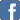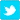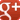Mole Concept - A Simple Way to Manipulate These Equations

Posted on the 12 March 2018 by Ewong0117
I was tutoring one of my students, and I realized she wasn't good at algebraic equation manipulation. She memorized the mole concept equations religiously. However, when the question requires her to do some manipulation to the equations she memorized, she struggles. While I told her the main solution to this problem is to improve her algebraic manipulation, I still came up with a simple solution to this, while she worked on improving her algebraic manipulation.

Triangle Method

The triangle method can be used when you have one of the following equations:(1) A = b x c(2) b = A / c
When given either of the above equation, put A at the top of the triangle and b and c at the bottom of the triangle.To find any of the variables at the bottom of the triangle, take the top divide by the either variable at the bottom of the triangle.
Hence you have (1) b = A / c  (2) c = A/ b
You will notice that the way the triangle is written, it looks like A/c or A/b, hence a way to memorize the above.
To find any of the variables at the top of the triangle, multiple the two variables at the bottom of the triangle together, i.e. A = b x c

Application of the Triangle Method to Mole Concept

Equation 1: Mole = Mass/ molar mass

You'll put Mass at the top of the triangle, and mole and molar mass at the bottom.The three equations you'll get out of this is
mass = molar mass x moles
molar mass = mass / moles
moles = mass / molar mass

Equation 2: Mole = Volume / Molar volume

You will put volume at the top of the triangle, and molar volume and moles at the bottom of the triangle:Note that this equation is only applicable for gases, and volume and molar volume must be in the same units. Example, if volume is in dm3, then molar volume must be in dm3.

Example 3: Concentration (g / dm3) = mass  (in g)/ volume (in dm3)

You will put mass at the top of the triangle, and concentration and volume at the bottom of the triangle.With that, you three equations you will get will be:
mass (g) = concentration (g/dm3) x volume (dm3)
concentration (g/ dm3) = mass (g) / volume (dm3)
volume (dm3) = mass (g)/ concentration (dm3)
Note that this equation can only be used for solutions (generally aqueous solutions for the O level syllabus).

Example 4: Concentration (mol / dm3) = moles/ volume (in dm3)

You will put moles at the top of the triangle, and concentration and volume at the bottom of the triangle.With that, you three equations you will get will be:
moles = concentration (mol/dm3) x volume (dm3)
concentration (mol/ dm3) = moles / volume (dm3)
volume (dm3) = moles/ concentration (dm3)
Note that this equation can only be used for solutions (generally aqueous solutions for the O level syllabus).

Example 5: Conversion between concentration in g/dm3 and mol/dm3

concentration (mol/dm3) = concentration (g/dm3) / molar mass
You will put concentration (g/dm3) at the top of the triangle, and concentration (mol/dm3) and volume (dm3) at the bottom of the triangle:concentration (g/dm3)  = concentration (mol/dm3) x molar mass
concentration (mol/ dm3) = concentration (g/dm3) /molar mass
molar mass =  concentration (g/dm3) /concentration (mol/ dm3)
Note that this equation can only be used for solutions (generally aqueous solutions for the O level syllabus).

Equation 6: Moles = Number of particles / Avogadro Constant

In this case, you will put number of particles at the top of the trianges. Moles and Avogadro Constant go to the bottom of the triangle.With that, the three equations you get are:number of particles = Avogadro's constant  x molesAvogadro constant = number of particles/ molesMoles = Number of particles / Avogadro constant.